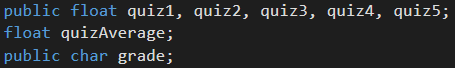For this challenge we’ll be making our program calculate 5 random numbers, take the average, and then based on the average, it will assign a letter grade. So if the average is 94, the grade will be an “A”, a 78 would be a “C”, etc.

Here’s the challenge details in pseudo code:

So we’ll be needing variables for the quiz numbers, the average, and the letter grade. Since the letter grade will be a single character, let’s use “char”. Here’s what I got:I made the scores and the char variables public so we can see the changes in the inspector.

While we do want to assign random numbers to the quizzes, we do want the numbers in a range from 0 to 100. So in the start class, our code will look like this:

Now we’ll want to add them up and divide by 5 to get the average.

Now its finally time for some fun stuff. To assign a letter grade, we’ll need to use some if statements. Let’s write some pseudo code to help clarify what conditions we need.

Now that we know what to do, let’s code it out. This is what I got:

If it works, it should look like this in the Unity inspector:

Aw well, it looks like Unity thought we didn’t do very well. But I think we got an A+